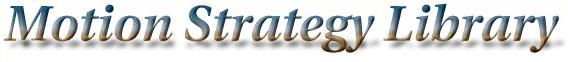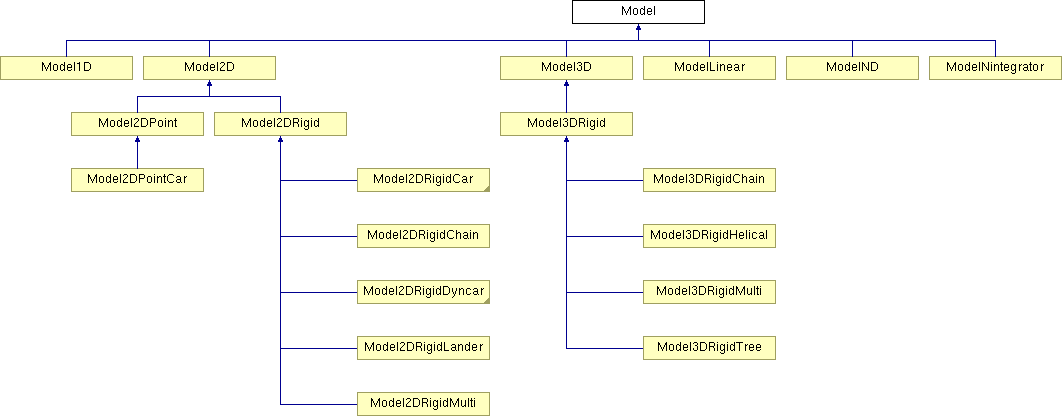Main Page   Class Hierarchy   Compound List   File List   Compound Members   File Members

# Model Class Reference

The incremental simulator model. More...

#include <model.h>

Inheritance diagram for Model::List of all members.

## Public Methods

Model (string path)
Empty constructor.

virtual ~Model ()
Empty destructor.

virtual list<MSLVectorGetInputs (const MSLVector &x)
Return a list of inputs, which may depend on state.

virtual MSLVector StateTransitionEquation (const MSLVector &x, const MSLVector &u)=0
The state transition equation, or equations of motion, xdot=f(x,u).

virtual bool Satisfied (const MSLVector &x)
Test whether global state-space constraints are satisfied.

virtual MSLVector Integrate (const MSLVector &x, const MSLVector &u, const double &h)=0
Perform integration from state x, using input u, over time step h.

virtual MSLVector LinearInterpolate (const MSLVector &x1, const MSLVector &x2, const double &a)
Linearly interpolate two state while respecting topology. More...

virtual MSLVector StateDifference (const MSLVector &x1, const MSLVector &x2)
Compute a MSLVector based on x2-x1. In R^n, the states are simply subtracted to make the MSLVector. This method exists to make things work correctly for other state-space topologies.

virtual MSLVector StateToConfiguration (const MSLVector &x)
A method that converts a Model state in to a Geom configuration.

virtual double Metric (const MSLVector &x1, const MSLVector &x2)
A distance metric, which is Euclidean in the base class.

virtual void Partialf_x (const MSLVector &x, const MSLVector &u, MSLMatrix &m)
Partial with respect to x of the state transition equation.

virtual void Partialf_u (const MSLVector &x, const MSLVector &u, MSLMatrix &m)
Partial with respect to u of the state transition equation.

virtual void L (const MSLVector &x, const MSLVector &u, double &l)
A cost or loss function, to be used in optimization problems.

virtual void PartialL_x (const MSLVector &x, const MSLVector &u, MSLMatrix &m)
Partial of the loss with respect to x.

virtual void PartialL_u (const MSLVector &x, const MSLVector &u, MSLMatrix &m)
Partial of the loss with respect to u.

virtual void Phi (const MSLVector &x, const MSLVector &u, const MSLVector &goalstate, double &phi)
The final-state cost or loss.

virtual void PartialPhi_x (const MSLVector &x, const MSLVector &u, const MSLVector &goalstate, MSLMatrix &m)
Partial of the final-state loss with respect to x.

virtual void PartialPhi_t (const MSLVector &x, const MSLVector &u, const MSLVector &goalstate, MSLMatrix &m)
Partial of the final-state loss with respect to u.

virtual void Psi (const MSLVector &x, const MSLVector &goalstate, MSLVector &psi)
An error MSLVector that compares a goal state to a given state.

virtual void PartialPsi_x (const MSLVector &x, const MSLVector &u, MSLMatrix &m)
Partial of the error MSLVector with respect to x.

virtual void PartialPsi_t (const MSLVector &x, const MSLVector &u, MSLMatrix &m)
Partial of the error MSLVector with respect to time.

## Public Attributes

string FilePath
This file path is used for all file reads.

MSLVector LowerState
MSLVector of minimum values for each state variable.

MSLVector UpperState
MSLVector of maximum values for each state variable.

MSLVector LowerInput
MSLVector of minimum values for each input variable.

MSLVector UpperInput
MSLVector of maximum values for each input variable.

int StateDim
The dimension of the state space.

int InputDim
The dimension of the input space.

## Protected Methods

MSLVector RungeKuttaIntegrate (const MSLVector &x, const MSLVector &u, const double &h)
Integrate xdot using 4th-order Runge-Kutta.

MSLVector EulerIntegrate (const MSLVector &x, const MSLVector &u, const double &h)
Integrate xdot using Euler integration.

## Protected Attributes

double ModelDeltaT
The time interval to use for numerical integration (affects accuracy).

list<MSLVectorInputs
The complete set of inputs.

## Detailed Description

The incremental simulator model.

The Model classes contain incremental simulators that model the kinematics and dynamics of a variety of mechanical systems. The methods allow planning algorithms to compute the future system state, given the current state, an interval of time, and a control input applied over that interval. (The planning algorithms select the appropriate inputs using this information.) Using object-oriented class derivations, a wide variety of simulators can be included.

## Constructor & Destructor Documentation

 Model::Model ( string path )
 Empty constructor.

 Model::~Model ( ) [inline, virtual]
 Empty destructor.

## Member Function Documentation

 MSLVector Model::EulerIntegrate ( const MSLVector & x, const MSLVector & u, const double & h ) [protected]
 Integrate xdot using Euler integration.

 list< MSLVector > Model::GetInputs ( const MSLVector & x ) [virtual]
 Return a list of inputs, which may depend on state.

 MSLVector Model::Integrate ( const MSLVector & x, const MSLVector & u, const double & h ) [pure virtual]
 Perform integration from state x, using input u, over time step h.

 void Model::L ( const MSLVector & x, const MSLVector & u, double & l ) [inline, virtual]
 A cost or loss function, to be used in optimization problems.

 MSLVector Model::LinearInterpolate ( const MSLVector & x1, const MSLVector & x2, const double & a ) [virtual]
 Linearly interpolate two state while respecting topology. If a=0, then x1 is returned; if a=1, then x2 is returned. All intermediate values of $a \in [0,1]$ yield intermediate states. This method is defined by Model.

 double Model::Metric ( const MSLVector & x1, const MSLVector & x2 ) [virtual]
 A distance metric, which is Euclidean in the base class.

 void Model::PartialL_u ( const MSLVector & x, const MSLVector & u, MSLMatrix & m ) [inline, virtual]
 Partial of the loss with respect to u.

 void Model::PartialL_x ( const MSLVector & x, const MSLVector & u, MSLMatrix & m ) [inline, virtual]
 Partial of the loss with respect to x.

 void Model::PartialPhi_t ( const MSLVector & x, const MSLVector & u, const MSLVector & goalstate, MSLMatrix & m ) [inline, virtual]
 Partial of the final-state loss with respect to u.

 void Model::PartialPhi_x ( const MSLVector & x, const MSLVector & u, const MSLVector & goalstate, MSLMatrix & m ) [inline, virtual]
 Partial of the final-state loss with respect to x.

 void Model::PartialPsi_t ( const MSLVector & x, const MSLVector & u, MSLMatrix & m ) [inline, virtual]
 Partial of the error MSLVector with respect to time.

 void Model::PartialPsi_x ( const MSLVector & x, const MSLVector & u, MSLMatrix & m ) [inline, virtual]
 Partial of the error MSLVector with respect to x.

 void Model::Partialf_u ( const MSLVector & x, const MSLVector & u, MSLMatrix & m ) [inline, virtual]
 Partial with respect to u of the state transition equation.

 void Model::Partialf_x ( const MSLVector & x, const MSLVector & u, MSLMatrix & m ) [inline, virtual]
 Partial with respect to x of the state transition equation.

 void Model::Phi ( const MSLVector & x, const MSLVector & u, const MSLVector & goalstate, double & phi ) [inline, virtual]
 The final-state cost or loss.

 void Model::Psi ( const MSLVector & x, const MSLVector & goalstate, MSLVector & psi ) [inline, virtual]
 An error MSLVector that compares a goal state to a given state.

 MSLVector Model::RungeKuttaIntegrate ( const MSLVector & x, const MSLVector & u, const double & h ) [protected]
 Integrate xdot using 4th-order Runge-Kutta.

 bool Model::Satisfied ( const MSLVector & x ) [virtual]
 Test whether global state-space constraints are satisfied.

 MSLVector Model::StateDifference ( const MSLVector & x1, const MSLVector & x2 ) [virtual]
 Compute a MSLVector based on x2-x1. In R^n, the states are simply subtracted to make the MSLVector. This method exists to make things work correctly for other state-space topologies.

 MSLVector Model::StateToConfiguration ( const MSLVector & x ) [virtual]
 A method that converts a Model state in to a Geom configuration.

 MSLVector Model::StateTransitionEquation ( const MSLVector & x, const MSLVector & u ) [pure virtual]
 The state transition equation, or equations of motion, xdot=f(x,u).

## Member Data Documentation

 string Model::FilePath
 This file path is used for all file reads.

 int Model::InputDim
 The dimension of the input space.

 list< MSLVector > Model::Inputs [protected]
 The complete set of inputs.

 MSLVector Model::LowerInput
 MSLVector of minimum values for each input variable.

 MSLVector Model::LowerState
 MSLVector of minimum values for each state variable.

 double Model::ModelDeltaT [protected]
 The time interval to use for numerical integration (affects accuracy).

 int Model::StateDim
 The dimension of the state space.

 MSLVector Model::UpperInput
 MSLVector of maximum values for each input variable.

 MSLVector Model::UpperState
 MSLVector of maximum values for each state variable.

The documentation for this class was generated from the following file: Motion Strategy Library

Web page maintained by Steve LaValle
Partial support provided by NSF CAREER Award IRI-970228 (LaValle), Honda Research.
Contributors: Anna Atramentov, Peng Cheng, James Kuffner, Steve LaValle, and Libo Yang.